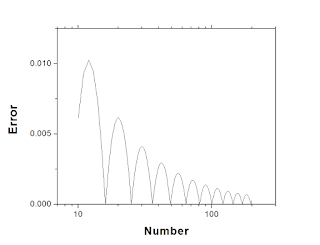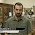## Friday, 6 November 2015

### A Trick for Mentally Approximating Square Roots

You won't believe this one simple trick for calculating square roots. Calculators hate me.

If you're the kind of person who needs to quickly calculate the square root of something, whether for finding out your crows-fly distance to a destination through a city grid, or determining whether the final score of your sports game was within statistical error or not, you might find this trick handy. It is not particularly advanced or arcane, it is just linear interpolation.

It requires you to know your perfect squares, to be able to do a quick subtraction in your head, and some even simpler addition. It is effective to the point that you can do these things.

Consider some number $S=Q^2$, and you want to find Q. Unless S is a perfect square, Q will be an irrational number, so any expression of it in terms of numbers will be an approximation. First find the integer N such that $N^{2}< S<(N+1)^{2}$. e.g. if S is 70, N is 8 because 70 is between $8^2$ and $9^2$. We approximate the irrational part of the square root, (Q-N), as a fraction found by linear interpolation. The denominator of the fraction will be the distance between the two perfect squares surrounding S, $(N+1)^{2}-N^{2}=2N+1$. The numerator is simply ($S-N^2$). Thus, to approximate the square root of a number, simply calculate:

$Q\approx N+\frac{S-N^{2}}{2N+1}$Demonstrating how this works for the square root of 70. The actual square root of 70 is marked off with a line.

So for our example of 70, N=8, S-N$^2$=70-64=6, 2N+1=17, so $Q\approx 8+\frac{6}{17}\approx 8.35$. This is roughly 0.2% away from the actual answer, about 8.36.Comparing the approximation to the actual square root function between 9 and 25. It's pretty close.

This operation basically assumes that square roots are distributed linearly between perfect squares. This is obviously not the case, but it gets more correct as the numbers get larger. By looking at the error, we can see that the approximation is within 1% for numbers larger than 10, and is worst when the fraction is $\frac{N}{2N+1}$. The worst-case for each perfect square interval decreases inversely with the number.The error associated with this approximation.
So how fast can this be done? For numbers below 100 it can be done mentally in one or two seconds, with practice. For bigger numbers, it'll probably take a bit longer. Obviously this gives you a fraction and not a decimal expansion. You can roughly guess a decimal expansion from the fraction, but that coarsens it a bit. In the above example, 6/17 is close to 6/18 so I could guess the decimal expansion is about .33.

Googling techniques for mental square roots, the first two are for finding the roots of large perfect squares, and then there is a Math Stack Exchange post about using a first-order Taylor expansion. This is more accurate because the Taylor series diverges as you approach the next perfect square, and I think this is faster as well.

1.I've been using this trick myself for estimating large square roots - e.g. SQRT(4235)

Shift the number down so it's roughly in the range 1-144, then estimate, and shift half way back up.

1.How do you "shift?"

2.Divide by powers of 100 (=drop digits at the end). Multiply the final result by powers of 10.

2.This comment has been removed by the author.

3.If you know N, wouldn't it be easier to consider the problem now to be finding a p such that (N + p)^2 = S. We know 0 < p < 1, so p^2 must be smaller than p, so we can approximate it as (N^2 + 2 N p) = S.

This gives us a new approximation, if we solve the equation for p. We can then iterate, which will give us another correction.

(This is actually Newton's method, and the number of correct digits will double each time we iterate.)

But I must admit, I like your method too :)

(Goodness, I hope this comment is formatted coherently!)

1.Can yo do it in your head?